### Home > CALC > Chapter 10 > Lesson 10.1.2 > Problem10-12

10-12.
1. Examine the integrals below. Consider the multiple tools available for integrating and use the best strategy. After evaluating each integral, write a short description of your method. Homework Help ✎

1.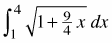2.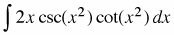3.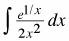4.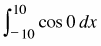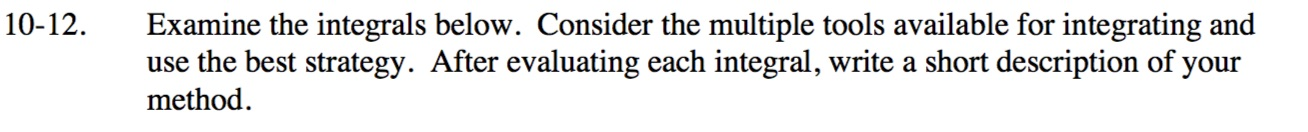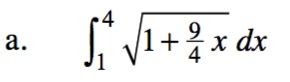$\int\Big(1+w\Big)^{1/2}dw=\frac{2}{3}\Big(1+w\Big)^{3/2}$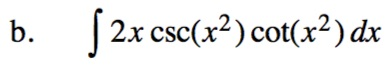$\frac{d}{dx}\csc(x)=-\csc(x)\cot(x)$

Use substitution. Let u = x2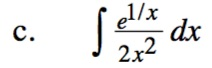Use substitution. Let u = 1/x.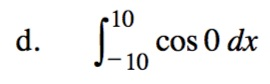What is the value of cos(0)?